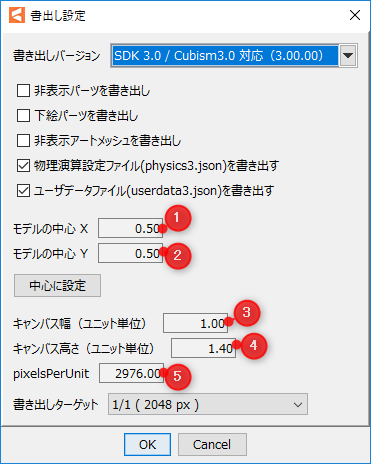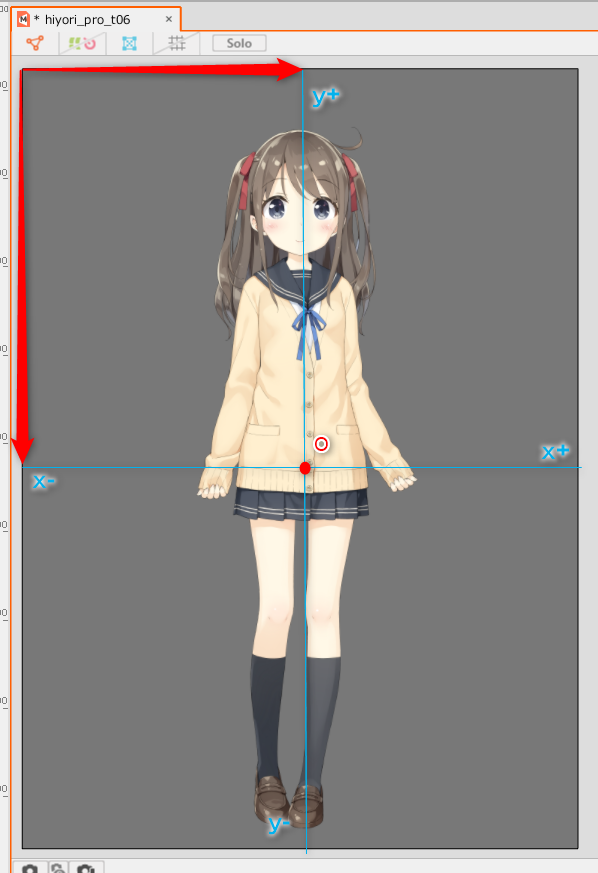# Range of DrawableVertexPositions

[Last updated : 11/07/2019]The model vertex information that can be retrieved from Cubsim Core for Native depends on the settings when outputting from the Editor. What is model vertex information? It refers to the XY coordinates obtained by the CubismModel::GetDrawableVertexPositions function in the Framework and the csmGetDrawableVertexPositions function in CubismCore. First, the origin is, in the dialog when saving, Center of model X (1) Center of model Y (2) value and is determined by the percentage distance from the top left to the whole.   Cubsim Core for Native uses the same coordinate system representation as OpenGL. The resulting vertex value is positive right up from the origin and is expressed in units indicated by (5). When dealing with right-handed coordinates, such as DirectX, the Y axis must be inverted. If the lower left corner is localX,localY in the Editor of the origin, the output coordinates will be X=(localX/(5))-((1) x (3)) Y=((2) x (4))-(localY / (5)) .     The range of values is For the x-axis, the range is from -(1) x (3) to (3) -((1) x (3)) . For the Y axis, the range is from ((2) x (4))-(4)  to  (2) x (4) . X(-0.5 to 0.5) Y(-0.7 to 0.7) if viewed in the upper left dialog© 2010 - 2022 Live2D Inc.